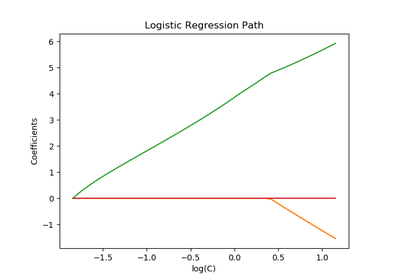# `sklearn.svm`.l1_min_c¶

`sklearn.svm.``l1_min_c`(X, y, loss=’squared_hinge’, fit_intercept=True, intercept_scaling=1.0)[source]

Return the lowest bound for C such that for C in (l1_min_C, infinity) the model is guaranteed not to be empty. This applies to l1 penalized classifiers, such as LinearSVC with penalty=’l1’ and linear_model.LogisticRegression with penalty=’l1’.

This value is valid if class_weight parameter in fit() is not set.

Parameters: X : array-like or sparse matrix, shape = [n_samples, n_features] Training vector, where n_samples in the number of samples and n_features is the number of features. y : array, shape = [n_samples] Target vector relative to X loss : {‘squared_hinge’, ‘log’}, default ‘squared_hinge’ Specifies the loss function. With ‘squared_hinge’ it is the squared hinge loss (a.k.a. L2 loss). With ‘log’ it is the loss of logistic regression models. ‘l2’ is accepted as an alias for ‘squared_hinge’, for backward compatibility reasons, but should not be used in new code. fit_intercept : bool, default: True Specifies if the intercept should be fitted by the model. It must match the fit() method parameter. intercept_scaling : float, default: 1 when fit_intercept is True, instance vector x becomes [x, intercept_scaling], i.e. a “synthetic” feature with constant value equals to intercept_scaling is appended to the instance vector. It must match the fit() method parameter. l1_min_c : float minimum value for C

## Examples using `sklearn.svm.l1_min_c`¶Path with L1- Logistic Regression